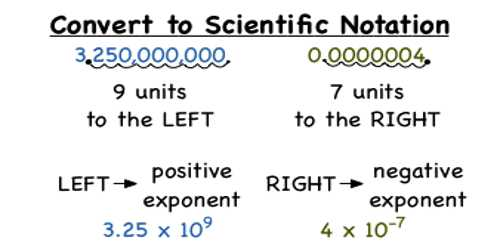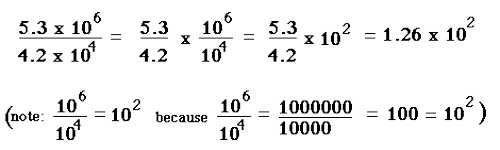Mathematic

# Converting Fractions to Scientific NotationConverting Fractions to Scientific Notation

Scientific notation is used to express very large or very small numbers. A number of scientific notation is written as the product of a number (integer or decimal) and a power of 10. The number has one digit to the left of the decimal point. The power of ten indicates how many places the decimal point was moved. Scientific notation is a standard way of writing very large and very small numbers so that they’re easier to both compare and use in computations.

Fractions are the Numbers which we can write in form of one number divided by another number. Here we express it as p/q, so we write ‘p’ as the numerator and ‘q’ as the denominator. Scientific notation is used to define the larger and smaller number. It is also written in the product form of Numbers or it is written in the power of 10 or in exponent form.For converting the fraction to scientific notation numbers the steps followed are:

• Take a number which is in the fraction form.
• Change the fraction number into the notation by putting the zeros present in the denominator in the exponent form or in the power of 10.
• If the denominator does not have any zeros then multiply both the numerator and denominator by a value so that it becomes a multiple of 10.

The fraction 6/1000000 written in scientific notation would be 6×10-6 because the denominator was decreased by 6 decimal places.

The fraction 65/1000000 written in scientific notation would be 6.5×10-5 because the denominator was decreased by 6 decimal places. One of the decimal places changed the numerator from 65 to 6.5.

A fraction smaller than 1 can be converted to scientific notation by decreasing the power of ten by one for each decimal place the denominator is decreased by.

Scientific notation numbers may be written in different forms. The number 6.5×10-7 could also be written as 6.5e-7.

Information Source: